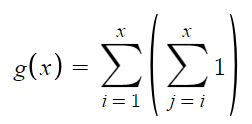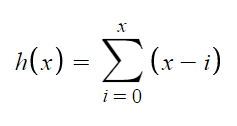Ask coding questions

← Back to all posts
##### [Solved] How to calcuate max number of possible substrings for a given string

For a given string, I'm looking for the most efficent way to calculate all substrings that can be created without rearranging or manipulating the string itself.

Let's say that I have the following string: "abc", 6 substrings can be created from it:
"a"
"b"
"c"
"ab"
"bc"
"abc"

That simple example should be extendable to any array, string, or iterable item.

After doing this for a few more examples, we end up with a small table that could be graphed:
String - number of substrings
"" - undefined
"a" - 1
"ab" - 3
"abc" - 6
"abcd" - 10
"abcde" - 15

Now, the contents of the string are irrelevant, all that matters is the item's length.

I was able to make a naive formula, given the length as x:When looking for performance, two loops isn't good... I was able to rewrite it to:which is marginally better, but I'm still looking for a single expression that might be able to replace the entire loop.

Interestingly, both of these graphs appear to be exponentional functions, they are higher than x^2, but less than 2^x.

Maybe there's still a better way to do this?

Answered by EpicGamer007 (1736) [earned 5 cycles]
View Answer
##### Comments
hotnewtop
EpicGamer007 (1736)

im sure you can search up find all substrings of string on google and get a faster algorithm

xxpertHacker (930)

@EpicGamer007 Dang, an answer on SO w/ no upvotes had the formula: (n * (n - 1) / 2).

EpicGamer007 (1736)

@xxpertHacker wow thats o(1) time i believe, can you link SO post?

EpicGamer007 (1736)

@xxpertHacker also, i was playing around with the formula and i think to get the total amount of substrings for a string with n length, the formula is actually ((n + 1) * n/2). Try it out with 5 for example or abcde

((5 + 1) * 5/2)
(6 * 5/2)
(30/2)
15

so I think the original formula gets the number of substrings for a string of length n - 1

xxpertHacker (930)

@EpicGamer007 Yes, I had already rewritten the formula exactly to the way that you had shown, since I graphed the formulas side-by-side and saw that the results were off by one.

https://www.desmos.com/calculator/ql9bqrr2kc

realTronsi (926)

@xxpertHacker Derivation is actually super easy and I think the key is thinking in terms of math and not code. I'll assume you have basic knowledge of probability and statistics, but then again you could be a 2nd grader who got held back in math for 6 years for all I know.

I'll explain my thinking:

Assuming "abc", any substring can be represented by selecting two indexes in the string. We can use n(n-1) as this will select two distinct indexes, but since single letters are also a substring, we can simply add n to make n(n-1) + n.

Now we can account for duplicates, which occur when stuff like this happens:

"a" then "b"
"b" then "a"

Both will result in "ab" as the final substring and the only difference is the order of which the letters are chosen. To fix this we can divide the non-single letter substrings by two.

n(n-1)/2 + n

# But anyways you don't even need to derive a formula for this if you just notice the pattern:

1, 3, 6, 10, 15

1 + 2 + 3 + 4 + 5

This is probably even easier to understand since you only need the intuition of a preschooler:

+1 is the number of substrings of length n
+2 is the number of substrings of length n-1
+3 is the number of substrings of length n-2

You can prove this through subtraction:

Suppose "abcde" and you were finding substrings of length n-1

You would have "abcd" then "bcde", essentially just shifting down until your current starting index is equal to n-1. Using this, we can derive it for n - x

N of substrings of len n - x = n - (n - x) + 1 = x + 1

So now that we know this, finding the sum of 1 + 2 + 3 ... + N is just n/2(1 + n) and holy shit would you look at that, what a surprise!

Conclusion: Programmers like to think recursively too much and xxpertHacker probably doesn't spend effort into his questions kek

Anyways yeah I kinda just Typescripted this Lisp of things on the spot SO if I stated something mathematically inaccurate feel free to Bash me about it since I am a bit Rusty. If you R confused about anything BASIC feel free to ASM me. Imma Swiftly Go now and get some Coffeescript so C you later.

^ I am very proud of my SQL and writing this is probably very Perl-lous because you're Going to Python me so Imma haskell into my Fortran.

^ I am so sorry

EpicGamer007 (1736)

@realTronsi lol. funny but good explanation.

xxpertHacker (930)

@realTronsi

Programmers like to think recursively too much

What about my thinking was recursive?

and xxpertHacker probably doesn't spend effort into his questions

a) That grammar is amazing
b) I didn't spend more than 5 minutes thinking about the problem, but by the wording in the post, I might trick the average bystander into thinking that I actually spent 6 minutes ;)

I just graphed it and gave up.

Btw, the entire conclusion is cringe.

xxpertHacker (930)

@realTronsi

knowledge of ...statistics

I never took statistics, I did calculus back when I should've been in it.

xxpertHacker (930)

@realTronsi

So now that we know this, finding the sum of 1 + 2 + 3 ... + N is just n/2(1 + n) and holy shit would you look at that, what a surprise!

ffs, I barely recognized that the expression is equivalent to (n + 1) choose 2, dang, I could've optimized this much faster.

realTronsi (926)

@xxpertHacker FFS I just typed a response and when I went to hit comment it clicked on your name instead. Oh well. Gimme a sec to retype everything

realTronsi (926)

@xxpertHacker too lazy to retype my essay response so here:

What about my thinking was recursive?

summations

a) That grammar is amazing

can't tell if sarcastic but theres nothing wrong with my grammar :D

b) I didn't spend more than 5 minutes thinking about the problem, but by the wording in the post, I might trick the average bystander into thinking that I actually spent 6 minutes ;)

u prolly could've solved it yourself easily in 10min rather than spending 30min chatting with kids in cyberspace

Btw, the entire conclusion is cringe.

That was the point, I was bored and wanted to annoy you which seemed to have worked :P

I never took statistics, I did calculus back when I should've been in it.

"never took" is this implying you finished hs/is your senior year?

ffs, I barely recognized that the expression is equivalent to (n + 1) choose 2, dang, I could've optimized this much faster.

I wasn't sure if you knew probability so I didn't use permu notations but using (n+1)C2 would be slower to calculate.

Also (n+1)C2 ??? I'm not sure I follow or I am being dumb rn. It should be nC2 + n unless that directly converts to (n+1)C2. Could you explain?

xxpertHacker (930)

@realTronsi

a) That grammar is amazing

can't tell if sarcastic but theres nothing wrong with my grammar :D

You said:

spend effort into his questions

You generally don't spend something "into" something else.

(n+1)C2 would be slower to calculate.

nCr(n + 1, 2) is equivalent to (n + 1)! / (n - 1)! * 2!, which is equivalent to (n + 1) * n / 2.

https://www.desmos.com/calculator/9xzuikject

realTronsi (926)

@xxpertHacker
Yes ik (n + 1)! / (n - 1)! * 2! = (n + 1) * n / 2

And now that I actually took time to calculate I see why they're equivalent, it just wasn't intuitive for me that nCr(n + 1, r) == nCr(n, r) + n

EDIT: nvm if I actually use my brain it is intuitive, bc the +n essentially adds a "layer", just like how (n+1) does, so nCr(n+x, r) = nCr(n, r) + i=1∑x(x-i+1)

also I've never had to type summations in text so sorry idk the format hopefully you can still read it

Also imma start using nCr() which is programmers' notation ig, since nCr is just ugly w/o latex

Also factorials would take more processing power

xxpertHacker (930)

@realTronsi Factorials would emit a loop, but for constant a k in nCr(t, k), the expression can always be unrolled. I was looking around for a generalized way of unrolling it, and Wikipedia had it: https://en.wikipedia.org/wiki/Binomial_coefficient#Binomial_coefficients_as_polynomials

realTronsi (926)

@xxpertHacker I thought you already knew that? But anyhow, solving factorials will always take some sort of loop/recursion, there is no shortcut. The only shortcut (which out brains can easily do but not computers) is by simplifying factorials on the num and denom, but using the (n + 1) * n / 2 formula will be faster anyhow.

If you can show me nCr in O(1) time I will gift you the noble prize and my life savings, which may or may not be \$0.

EDIT: Also no using quantum computers

xxpertHacker (930)

@realTronsi I was going to consider a hardware implementation that computes the value in parallel, but then why do that?

I could just search it up on the web, well, here we go, SO: https://stackoverflow.com/a/37779107

Also, a static table could be used instead.

realTronsi (926)

@xxpertHacker Okay that's technically cheating because you're using an approximation... also you could use the table argument anywhere, I could just have a table of every single possible operation result and use a hash table to solve any mathematical equation in O(1)

xxpertHacker (930)

@realTronsi Eh, is it really cheating? All floating point math in computers is approximated, since there's a limit on max/min values, and not infinite precision.